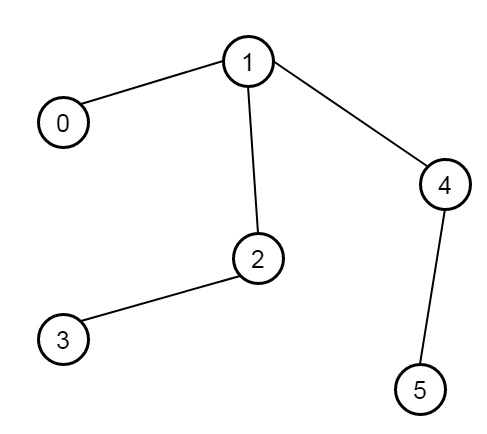# Tree Diameter in C++

C++Server Side ProgrammingProgramming

Suppose we have an undirected tree; we have to find its diameter − the number of edges in the longest path in that tree is the diameter of that tree. Here tree is given as an edge list where edges[i] = [u, v] is a bidirectional edge between nodes u and v. Each node has labels in the set {0, 1, ..., edges.length}. So if the graph is like −The output will be 4.

To solve this, we will follow these steps −

• Define a map l
• define a method called dfs(). this will take v, an array called visited, the graph and c. It will work as follows −
• visited[v] := true, set ans := 0
• for i in range 0 to size of graph[v]
• if visited[graph[v, i]] is false, then
• ans := max of ans, dfs(graph[v, i], visited, graph, c + 1)
• if c > best, then best := c and node := v
• set visited[v] := false
• return max of c and ans
• In the main method, it will take the edge list e
• n := size of e, make an array called graph of size n + 1
• for i in range 0 to n – 1
• insert e[i, 1] into graph[e[i, 0]] and insert e[i, 0] into graph[e[i, 1]]
• make two arrays visited, and visited2 array of size n + 1, set best := 0 and node := 0
• call dfs(0, visited, graph)
• return dfs(node, visited2, graph)

## Example(C++)

Let us see the following implementation to get a better understanding −

Live Demo

#include <bits/stdc++.h>
using namespace std;
#define pb push_back
class Solution {
public:
map <int ,int > l;
int best;
int node;
int dfs(int v, bool* visited, vector <int> graph[], int c = 0){
visited[v] = true;
int ans = 0;
for(int i = 0; i < graph[v].size(); i++){
if(!visited[graph[v][i]])ans = max(ans,dfs(graph[v][i], visited, graph, c+1));
}
if(c > best){
best = c;
node = v ;
}
visited[v] = false;
return max(c,ans);
}
int treeDiameter(vector<vector<int>>& e) {
int n = e.size();
vector <int> graph[n+1];
for(int i = 0; i < n; i++){
graph[e[i]].pb(e[i]);
graph[e[i]].pb(e[i]);
}
bool* visited = new bool[n+1]();
best = 0;
node = 0;
dfs(0, visited, graph);
bool* visited2 = new bool[n+1]();
return dfs(node, visited2, graph);
}
};
main(){
vector<vector<int>> v = {{0,1},{1,2},{2,3},{1,4},{4,5}};
Solution ob;
cout <<ob.treeDiameter(v);
}

## Input

[[0,1],[1,2],[2,3],[1,4],[4,5]]

## Output

4